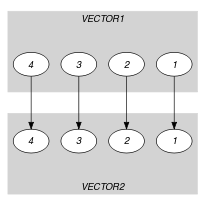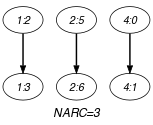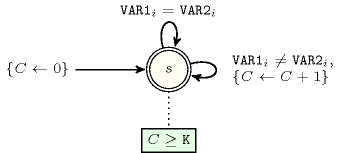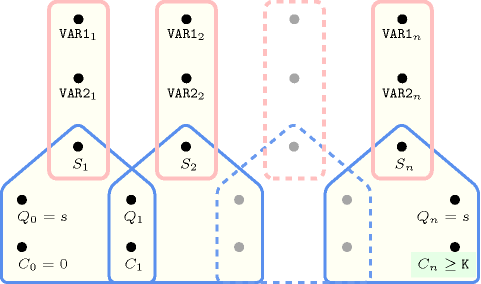## 5.115. differ_from_at_least_k_pos

Origin

Inspired by [Frutos97].

Constraint

$\mathrm{𝚍𝚒𝚏𝚏𝚎𝚛}_\mathrm{𝚏𝚛𝚘𝚖}_\mathrm{𝚊𝚝}_\mathrm{𝚕𝚎𝚊𝚜𝚝}_𝚔_\mathrm{𝚙𝚘𝚜}\left(𝙺,\mathrm{𝚅𝙴𝙲𝚃𝙾𝚁}\mathtt{1},\mathrm{𝚅𝙴𝙲𝚃𝙾𝚁}\mathtt{2}\right)$

Type
 $\mathrm{𝚅𝙴𝙲𝚃𝙾𝚁}$ $\mathrm{𝚌𝚘𝚕𝚕𝚎𝚌𝚝𝚒𝚘𝚗}\left(\mathrm{𝚟𝚊𝚛}-\mathrm{𝚍𝚟𝚊𝚛}\right)$
Arguments
 $𝙺$ $\mathrm{𝚒𝚗𝚝}$ $\mathrm{𝚅𝙴𝙲𝚃𝙾𝚁}\mathtt{1}$ $\mathrm{𝚅𝙴𝙲𝚃𝙾𝚁}$ $\mathrm{𝚅𝙴𝙲𝚃𝙾𝚁}\mathtt{2}$ $\mathrm{𝚅𝙴𝙲𝚃𝙾𝚁}$
Restrictions
 $|\mathrm{𝚅𝙴𝙲𝚃𝙾𝚁}|\ge 1$ $\mathrm{𝚛𝚎𝚚𝚞𝚒𝚛𝚎𝚍}$$\left(\mathrm{𝚅𝙴𝙲𝚃𝙾𝚁},\mathrm{𝚟𝚊𝚛}\right)$ $𝙺\ge 0$ $𝙺\le |\mathrm{𝚅𝙴𝙲𝚃𝙾𝚁}\mathtt{1}|$ $|\mathrm{𝚅𝙴𝙲𝚃𝙾𝚁}\mathtt{1}|=|\mathrm{𝚅𝙴𝙲𝚃𝙾𝚁}\mathtt{2}|$
Purpose

Enforce two vectors $\mathrm{𝚅𝙴𝙲𝚃𝙾𝚁}\mathtt{1}$ and $\mathrm{𝚅𝙴𝙲𝚃𝙾𝚁}\mathtt{2}$ to differ from at least $𝙺$ positions.

Example
$\left(2,〈2,5,2,0〉,〈3,6,2,1〉\right)$

The $\mathrm{𝚍𝚒𝚏𝚏𝚎𝚛}_\mathrm{𝚏𝚛𝚘𝚖}_\mathrm{𝚊𝚝}_\mathrm{𝚕𝚎𝚊𝚜𝚝}_𝚔_\mathrm{𝚙𝚘𝚜}$ constraint holds since the first and second vectors differ from 3 positions, which is greater than or equal to $𝙺=2$.

Typical
 $𝙺>0$ $𝙺<|\mathrm{𝚅𝙴𝙲𝚃𝙾𝚁}\mathtt{1}|$ $|\mathrm{𝚅𝙴𝙲𝚃𝙾𝚁}\mathtt{1}|>1$
Symmetries
• Arguments are permutable w.r.t. permutation $\left(𝙺\right)$ $\left(\mathrm{𝚅𝙴𝙲𝚃𝙾𝚁}\mathtt{1},\mathrm{𝚅𝙴𝙲𝚃𝙾𝚁}\mathtt{2}\right)$.

• $𝙺$ can be decreased to any value $\ge 0$.

• Items of $\mathrm{𝚅𝙴𝙲𝚃𝙾𝚁}\mathtt{1}$ and $\mathrm{𝚅𝙴𝙲𝚃𝙾𝚁}\mathtt{2}$ are permutable (same permutation used).

Arg. properties

Extensible wrt. $\mathrm{𝚅𝙰𝚁𝙸𝙰𝙱𝙻𝙴𝚂}\mathtt{1}$ and $\mathrm{𝚅𝙰𝚁𝙸𝙰𝙱𝙻𝙴𝚂}\mathtt{2}$ (add items at same position).

Remark

Used in the Arc constraint(s) slot of the $\mathrm{𝚊𝚕𝚕}_\mathrm{𝚍𝚒𝚏𝚏𝚎𝚛}_\mathrm{𝚏𝚛𝚘𝚖}_\mathrm{𝚊𝚝}_\mathrm{𝚕𝚎𝚊𝚜𝚝}_𝚔_\mathrm{𝚙𝚘𝚜}$ constraint.

Used in

implied by: $\mathrm{𝚍𝚒𝚏𝚏𝚎𝚛}_\mathrm{𝚏𝚛𝚘𝚖}_\mathrm{𝚎𝚡𝚊𝚌𝚝𝚕𝚢}_𝚔_\mathrm{𝚙𝚘𝚜}$ ($\ge 𝙺$ replaced by $=𝙺$).

Keywords
Arc input(s)

$\mathrm{𝚅𝙴𝙲𝚃𝙾𝚁}\mathtt{1}$ $\mathrm{𝚅𝙴𝙲𝚃𝙾𝚁}\mathtt{2}$

Arc generator
$\mathrm{𝑃𝑅𝑂𝐷𝑈𝐶𝑇}$$\left(=\right)↦\mathrm{𝚌𝚘𝚕𝚕𝚎𝚌𝚝𝚒𝚘𝚗}\left(\mathrm{𝚟𝚎𝚌𝚝𝚘𝚛}\mathtt{1},\mathrm{𝚟𝚎𝚌𝚝𝚘𝚛}\mathtt{2}\right)$

Arc arity
Arc constraint(s)
$\mathrm{𝚟𝚎𝚌𝚝𝚘𝚛}\mathtt{1}.\mathrm{𝚟𝚊𝚛}\ne \mathrm{𝚟𝚎𝚌𝚝𝚘𝚛}\mathtt{2}.\mathrm{𝚟𝚊𝚛}$
Graph property(ies)
$\mathrm{𝐍𝐀𝐑𝐂}$$\ge 𝙺$

Graph model

Parts (A) and (B) of Figure 5.115.1 respectively show the initial and final graph associated with the Example slot. Since we use the $\mathrm{𝐍𝐀𝐑𝐂}$ graph property, the arcs of the final graph are stressed in bold.

##### Figure 5.115.1. Initial and final graph of the $\mathrm{𝚍𝚒𝚏𝚏𝚎𝚛}_\mathrm{𝚏𝚛𝚘𝚖}_\mathrm{𝚊𝚝}_\mathrm{𝚕𝚎𝚊𝚜𝚝}_𝚔_\mathrm{𝚙𝚘𝚜}$ constraint(a) (b)
Automaton

Figure 5.115.2 depicts the automaton associated with the $\mathrm{𝚍𝚒𝚏𝚏𝚎𝚛}_\mathrm{𝚏𝚛𝚘𝚖}_\mathrm{𝚊𝚝}_\mathrm{𝚕𝚎𝚊𝚜𝚝}_𝚔_\mathrm{𝚙𝚘𝚜}$ constraint. Let $\mathrm{𝚅𝙰𝚁}{\mathtt{1}}_{i}$ and $\mathrm{𝚅𝙰𝚁}{\mathtt{2}}_{i}$ be the ${i}^{th}$ variables of the $\mathrm{𝚅𝙴𝙲𝚃𝙾𝚁}\mathtt{1}$ and $\mathrm{𝚅𝙴𝙲𝚃𝙾𝚁}\mathtt{2}$ collections. To each pair of variables $\left(\mathrm{𝚅𝙰𝚁}{\mathtt{1}}_{i},\mathrm{𝚅𝙰𝚁}{\mathtt{2}}_{i}\right)$ corresponds a signature variable ${S}_{i}$. The following signature constraint links $\mathrm{𝚅𝙰𝚁}{\mathtt{1}}_{i}$, $\mathrm{𝚅𝙰𝚁}{\mathtt{2}}_{i}$ and ${S}_{i}$: $\mathrm{𝚅𝙰𝚁}{\mathtt{1}}_{i}=\mathrm{𝚅𝙰𝚁}{\mathtt{2}}_{i}⇔{S}_{i}$.

##### Figure 5.115.2. Automaton of the $\mathrm{𝚍𝚒𝚏𝚏𝚎𝚛}_\mathrm{𝚏𝚛𝚘𝚖}_\mathrm{𝚊𝚝}_\mathrm{𝚕𝚎𝚊𝚜𝚝}_𝚔_\mathrm{𝚙𝚘𝚜}$ constraint##### Figure 5.115.3. Hypergraph of the reformulation corresponding to the automaton of the $\mathrm{𝚍𝚒𝚏𝚏𝚎𝚛}_\mathrm{𝚏𝚛𝚘𝚖}_\mathrm{𝚊𝚝}_\mathrm{𝚕𝚎𝚊𝚜𝚝}_𝚔_\mathrm{𝚙𝚘𝚜}$ constraint